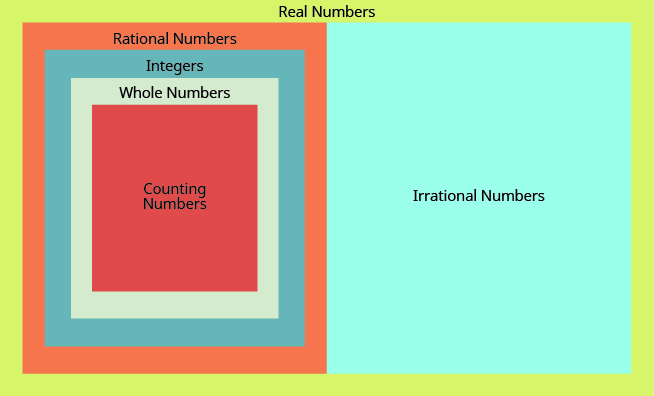## Key Concepts

• Real numbers## Glossary:

Irrational number
A number that cannot be written as the ratio of two integers. Its decimal form does not stop and does not repeat.
Rational number
A number that can be written in the form ${\Large\frac{p}{q}}$ , where p and q are integers and $q\ne 0$ . Its decimal form stops or repeats.
Real number:
A number that is either rational or irrational.